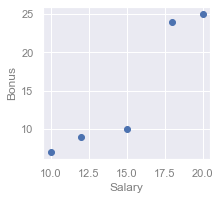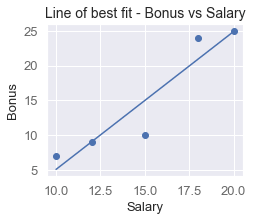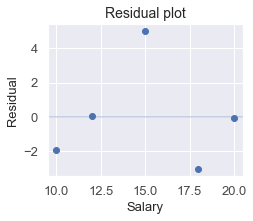search
Search
Map of Data Science
Guest 0reps
exit_to_appLog out
Map of data science
Thanks for the thanks!
close
chevron_left Linear Regression
Cancel
Post
account_circle
Profile
exit_to_app
Sign out
search
keyboard_voice
close
Searching Tips
Search for a recipe:
"Creating a table in MySQL"
Search for an API documentation: "@append"
Search for code: "!dataframe"
Apply a tag filter: "#python"
Useful Shortcuts
/ to open search panel
Esc to close search panel
to navigate between search results
d to clear all current filters
Enter to expand content previewDoc SearchCode Search BetaSORRY NOTHING FOUND!
mic
Start speaking...Voice search is only supported in Safari and Chrome.
Shrink
Navigate to
A
A
brightness_medium
share
arrow_backShareTwitterFacebook
chevron_left Linear Regression
check_circle
Mark as learned
thumb_up
0
thumb_down
0
chat_bubble_outline
0
auto_stories new
settings

# Linear regression using Scikit-learn

Machine Learning
chevron_right
Linear Regression
schedule Jul 1, 2022
Last updated
local_offer
Tags
map
Check out the interactive map of data science

This is a tutorial about implementing linear regression using Python's Sciki-learn library. To learn about the theory behind linear regression, check out our tutorial here.

# Introduction

Consider the following dataset about the income of 5 employees:

Salary

Position

Bonus

20

Manager

25

18

Manager

24

10

Staff

6

12

Staff

9

15

Staff

10

Here, our goal is to predict the bonus of an employee using their salary and position using linear regression. This means that salary and position are our predictor variables and features, and bonus is our target variable. For linear regression, we should also be mindful about the type of the variables:

• salary is continuous

• position is categorical

Traditionally, linear regression is performed on continuous predictor variables like salary, but the algorithm can be adapted to handle categorical variables as well. To keep things simple, let us first predict the bonus solely based off the salary.

# Data visualisation

Here's a quick visualisation of our dataset:Mathematically, our first goal is to find the optimal weights, $\theta_0$ and $\theta_1$, of a linear curve:

$$y=\theta_0 +\theta_1 x_1$$

Where,

• $y$ is bonus

• $x_1$ is salary

• $\theta_0$ and $\theta_1$ are the weights (intercept and slope) we wish to optimise

# Implementation

## Preprocessing

Let's first begin by creating our data-set:

 import numpy as npsalary = np.array([20,18,10,12,15])bonus = np.array([25,24,7,9,10]) 

Here, salary and bonus are both a simple 1D Numpy array. However, in order to make them compatible with Scikit-learn's models, we must first convert them to 2D arrays like so:

 X_salary = salary.reshape(len(salary),1)X_salary array([, , , , ]) 

Similarly, converting our bonus array to a 2D array:

 y_bonus = bonus.reshape(len(bonus),1)y_bonus array([, , [ 7], [ 9], ]) 

That's it for the preprocessing stage.

## Training our model

We can now directly perform linear regression using Scikit-learn:

 from sklearn.linear_model import LinearRegressionmodel = LinearRegression()model.fit(x_salary, y_bonus)print("Slope:", model._coef)print("Intercept:", model._intercept) Slope: 1.9852941176470584Intercept: -14.779411764705877 

This means that the equation for the line of best fit is as follows:

$$\hat{y}=-1.99x_1+14.78$$

Where,

• $\hat{y}$ is the predicted bonus of an employee

• $x_1$ is the salary of an employee

## Visualising our model

Since we now have our optimal weights, we can easily graph our linear model:

 x = np.linspace(10,20)y_predictions = model.predict(x.reshape(-1,1))plt.scatter(x_salary, y_bonus)plt.plot(x, y_predictions)plt.xlabel("Salary")plt.ylabel("Bonus")plt.title("Line of best fit - Bonus vs Salary")plt.show() 

This produces the following plot:We can see that our model reasonably strikes through the center of our data points.

## Performing predictions

To predict employees' bonus given their salary, use the predict(~) method we saw earlier:

 x_to_predict = np.array([13,16])model.predict(x_to_predict.reshape(-1,1)) array([[11.02941176], [16.98529412]]) 

The output tells us that:

• an employee with salary=13 would have a bonus of roughly 11.

• an employee with salary=16 would have a bonus of roughly 17.

## Evaluating performance

### Plotting residuals

Residuals tell us how off each of our predictions is, and it is mathematically defined as follows:

$$r^{(i)}=y^{(i)}-\hat{y}^{(i)}$$

Where,

• $r^{(i)}$ is the residual of the $i$-th data point

• $y^{(i)}$ is the true value of the $i$-th data point

• $\hat{y}^{(i)}$ is the predicted value of the $i$-th data point

A high residual for a prediction means that our model did a terrible job at predicting.

We typically use residual plots to visualise all the residuals:

 plt.xlabel("Salary", fontsize=13)plt.ylabel("Residual", fontsize=13)plt.title("Residual plot", fontsize=14)y_predictions = model.predict(x_salary)plt.scatter(x_salary, y_predictions - y_bonus)plt.axhline(0, alpha=0.3)plt.show() 

This produces the following:### Mean squared error

Recall from our tutorial covering the theory of linear regression, the mean squared error (MSE) measures how off, on average, our predicted values are:

$$\mathrm{MSE}=\frac{1}{n}\sum^n_{i=1}\left(y^{(i)}-\hat{y}^{(i)} \right)^2$$

Where,

• $n$ is the size of our dataset ($5$ in this case)

• $y^{(i)}$ is the true value of the $i$-th data point

• $\hat{y}^{(i)}$ is the predicted value of the $i$-th data point

The MSE comes in handy when you want to compare between different linear regression models. For instance, suppose you fit a polynomial curve instead of a linear curve as we did here, and you want to know which of the two fits the data better. We can compute their respective MSE, and the model with the lower MSE is the one that has the tighter fit.

We can compute the MSE easily using Scikit-learn's built in method:

 from sklearn.metrics import mean_squared_errorprint("MSE:", mean_squared_error(x_salary, y_bonus)) MSE: 20.8